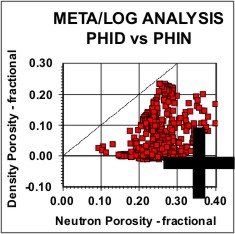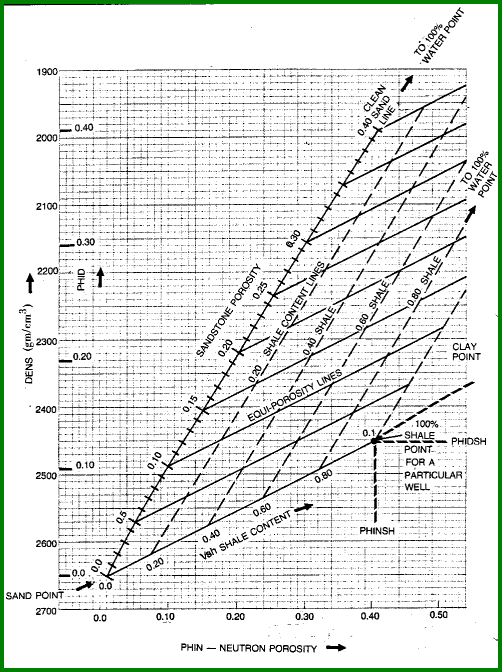Shale Volume from Density Neutron Crossplot
The separation between neutron and density porosity is a common method for calculating shale volume. It is accurate only when the shaly sand contains pure quartz plus clay minerals. Most sandstones are not pure quartz.  When other minerals are present, some of the separation is due to these minerals. Micas, siderite, ankerite, and volcanic rock fragments are common in many sandstones. All are heavier than quartz, causing the excess separation by reducing density porosity and increasing neutron porosity.

The response equation for the density log in porosity units follows the classical form:

1: PHID = PHIe * Sxo * PHIDw (water term)
+ PHIe * (1 - Sxo) * PHIDh (hydrocarbon term)
+ Vsh * PHIDsh (shale term)
+ (1 - Vsh - PHIe) * Sum (Vi * PHIDi) (matrix term)

Where:
PHIDh = log reading in 100% hydrocarbon
PHIDi = log reading in 100% of the ith component of matrix rock
PHIDsh = log reading in 100% shale
PHIDw = log reading in 100% water
PHIe = effective porosity (fractional)
Sxo = water saturation in invaded zone (fractional)
Vi = volume of ith component of matrix rock
Vsh = volume of shale (fractional)

The response equation for the neutron porosity log also follows the classical form:

2: PHIN = PHIe * Sxo * PHINw (water term)
+ PHIe * (1 - Sxo) * PHINh (hydrocarbon term)
+ Vsh * PHINsh (shale term)
+ (1 - Vsh - PHIe) * Sum (Vi * PHINi) (matrix term)

Where:
PHINh = log reading in 100% hydrocarbon
PHINi = log reading in 100% of the ith component of matrix rock
PHINsh = log reading in 100% shale
PHINw = log reading in 100% water
PHIe = effective porosity (fractional)
Sxo = water saturation in invaded zone (fractional)
Vi = volume of ith component of matrix rock
Vsh = volume of shale (fractional)Shale Content from Density Neutron
PHIDw = PHIDh = PHINw = PHINh = 1.0, PHIDi = PHINi = 0.0. Sxo = 1.0

Then by subtracting the two equations and solving for Vsh, we get:

3: VSHxnd = (PHIN - PHID) / (PHINSH - PHIDSH)
4:  VSHxnd = Min(1, Max(0, VSHxnd))

Thus, the algebraic formula to solve for shale volume from the density neutron crossplot is a linear interpolation of the separation between the density and neutron porosity log curves.

5: VSHxnd = (PHIN - PHID) / (PHINSH - PHIDSH)

Where:
PHID = density log porosity reading in zone of interest (fractional)
PHIDSH = apparent density porosity in shale (fractional)
PHIN = neutron log reading in zone of interest fractional)
PHINSH = neutron log reading in 100% shale (fractional)
VSHxnd = shale volume from density neutron crossplot (fractional)COMMENTS:
Shale volume from the density neutron crossplot should only be attempted in oil or water bearing shaly sands, not in dolomite, anhydrite, or gas zones. This is because the separation between the two curves is not a function of shale in these cases. The density neutron will help resolve shale volume in radioactive sands (like granite wash formations) provided the zone is known to be sandstone. It will not help resolve a radioactive dolomite.If the density neutron data is recorded in percentage units instead of fractional units, convert the data to fractions by dividing each value by 100. If percentage data is used in error, the results will still be correct, but Vsh will be in fractional units.RECOMMENDED PARAMETERS:
Range            Default
PHIDSH     -0.03 to +0.10       0.00
PHINSH       0.10 to 0.40        0.30
Choose from crossplot or depth plot.NUMERICAL EXAMPLE:
Assume PHID = 0.12, PHIN = 0.28, PHIDSH = 0.03, PHINSH = 0.30
Vsh from density neutron crossplot:
VSHxnd = (0.28 - 0.12) / (0.30 - 0.03) = 0.59Shale Content from Density Neutron with Matrix Offset
The previous method described for density neutron data assumes the clean line for the crossplot is identical to the units of the density neutron log (i.e. sandstone or limestone). If you wish the clean line to be at a matrix value other than that for the log units, both neutron and density data must be shifted to account for this matrix change.

A chart illustrating the construction of the shale content lines on a density neutron crossplot is given below. This is the normal density neutron crossplot for determination of porosity and shale volume in the shaly sand model when the sand fraction is made up of pure quartz.Shale Volume from Density Neutron Crossplot

A matrix offset is needed if the sand contains heavy minerals in place of some or all of the quartz. The matrix offset translates the origin along a 45 degree line for CNL data, and at a steeper angle for SNP data. This is equivalent to the solution of the two response equations described earlier, with PHIDi and PHINi not equal to zero, but determined from knowledge of the actual rock description.

Reconstitute density data from density porosity log.
6: DENS = PHID * KD1 + (1 - PHID) * KD2

Where:
KD1 = 1.00 gm/cc English units   1000 kg/m3 Metric Units
KD2 = 2.65 gm/cc English units   2650 kg/m3 Metric Units

Calculate density porosity for desired matrix and fluid values.
7: PHIDm = (DENSMA - DENS) / (DENSMA - DENSW)

Calculate density offset for this matrix and fluid.
8: D = PHIDm - PHID

Calculate neutron offset for same matrix.
9: C = KN4 * D

Where:
KN4 = 1.00 for CNL   0.75 for SNP neutron log

Calculate neutron log reading for same matrix.
10: PHINm = PHIN - C

11: PHIDSHm = PHIDSH + D
12: PHINSHm = PHINSH - C

Calculate shale content.
13: VSHxm = (PHINm - PHIDm) / (PHINSHm - PHIDSHm)
14:  VSHxm = Min(1, Max(0, VSHxm))

Where:
C = neutron log offset (fractional)
D = density log offset (fractional)
DENS = density log reading (kg/m3 or gm/cc)
PHID = density log reading (fractional)
PHIDm = density log reading in zone of interest (fractional)
PHIDSH = apparent density porosity in shale (fractional)
PHIDSHm = density log reading in 100% shale in zone of interest (fractional)
PHIN = neutron log reading in zone of interest (fractional)
PHINm = neutron log reading correction for matrix offset (fractional)
PHINSH = neutron log reading in 100% shale fractional)
PHINSHm = neutron log reading in 100% shale corrected for matrix offset (fractional)
DENSMA = matrix density (kg/m3 or gm/cc)
DENSW = fluid density (kg/m3 or gm/cc)
VSHxm = shale volume from density neutron crossplot corrected for matrix offset (fractional)COMMENTS:
If density log is in density units, skip Step 1.RECOMMENDED PARAMETERS:
Range          Default
PHIDSH    -0.03 to +0.10       0.00
PHINSH      0.10 to 0.40        0.30NUMERICAL EXAMPLE:
Using data for Sand "D".
PHID = 0.12
PHIDSH = 0.03
PHIN = 0.28
PHINSH = 0.30
Units = Sandstone
Desired DENSMA = 2740 kg/m3

DENS = (0.12 * 1000 + (1 - 0.12) * (2650)) = 2452 kg/m3
PHIDm = (2740 - 2452) / (2740 - 1000) = 0.165
D = 0.165 - 0.12 = + 0.045
C = - 0.045
PHINm = 0.28 - 0.045 = 0.235
PHIDSHm = 0.03 + 0.045 = 0.075
PHINSHm = 0.30 - 0.045 = 0.255
VSHxm = (0.235 - 0.165) / (0.233 = 0.075) = 0.39

This result agrees more closely with GR and SP data and suggests the matrix offset was reasonable and necessary. The value with no offset applied was 0.59 from the previous example.

Page Views ---- Since 01 Jan 2015